# Test: Introduction To Determinants

## 10 Questions MCQ Test Mathematics (Maths) Class 12 | Test: Introduction To Determinants

Description
Attempt Test: Introduction To Determinants | 10 questions in 10 minutes | Mock test for JEE preparation | Free important questions MCQ to study Mathematics (Maths) Class 12 for JEE Exam | Download free PDF with solutions
QUESTION: 1

### Order of a matrix [ 2 5 7 ] is:

Solution:

The order of matrix is defined by (row × column). The follwing matrix has 1 row and 3 columns. So, the correct option is (d) i.e 1×3.

QUESTION: 2

### The value of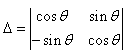Solution:

To find the determinant we have to cross mutliply the elements and then subtract it.
cos2θ - (-sin2θ) = 1

QUESTION: 3

### If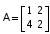, then the value of |2A| is same as​:

Solution:

Due to the rule if a square matrix is of order n x n then, |kA| = kn |A|.

QUESTION: 4

Value of the determinant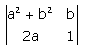Solution:

(a2 + b2)×1 - (2a) × b
= a2 +b2 - 2ab
= (a - b)2

QUESTION: 5

​If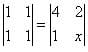, then the value of x is:

Solution:

As value of both determinants are equal
∴ (1×1) - (1×1) = 4x - 2
0 = 4x-2.
4x = 2.
x = 2/4 = 1/2

QUESTION: 6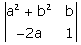​is equal to

Solution:

Determinant = [(a2 + b2).1 - (-2ab)]
= (a+b)2

QUESTION: 7

If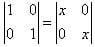, then the value of x is  ​

Solution:

As the value of both determinants are equal,
∴ 1 = x2
x = ±1

QUESTION: 8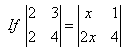, then the value of x is​

Solution:

8-6 = 4x-2x
⇒ x = 1

QUESTION: 9

The value of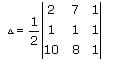is​

Solution:

Δ = 1/2 [2(1-8) -7(1-10) +1(8-10)]
= 47/2

QUESTION: 10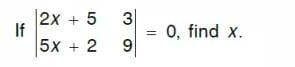Solution:

9(2x+5) - 3(5x+2) = 0
3x = -39
x = -13Use Code STAYHOME200 and get INR 200 additional OFF Use Coupon Code